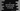# C program to print the length of each words in a string## Introduction :

In this tutorial, we will learn how to print the length of each words in a string in C. Our program will take one string as input from the user and print out each word with its length. This program will give you an idea on how to read user input string, how to iterate through the characters of that string and how to print one substring of that string.

We will iterate the string only once i.e. the complexity of the program will be O(n).

## C program to find length of each words in a string :

``````#include <stdio.h>
#include <string.h>

// 1
#define STRING_MAX_SIZE 50

int main()
{
// 2
char givenString[STRING_MAX_SIZE];

// 3
printf("Enter a string : ");
fgets(givenString, STRING_MAX_SIZE, stdin);

// 4
int i;
int endIndex;
int startIndex = 0;

// 5
for (i = 0; i < strlen(givenString); i++)
{
// 6
if (givenString[i] == ' ' || i == strlen(givenString) - 1)
{
// 7
endIndex = i;
printf("%.*s = %d\n", (endIndex - startIndex), givenString + startIndex, (endIndex - startIndex));
startIndex = i + 1;
}
}

return 0;
}``````

### Explanation :

The commented numbers in the above program denote the step numbers below :

1. Define one variable STRINGMAXSIZE as 50. We will use it as the maximum size of a string it can store.
2. givenString is a character array of size STRINGMAXSIZE. This array will hold the user input string.
3. fgets is used to read a string in C. Ask the user to enter a string, read and store it in givenString.
4. Create three integer variables : i to use in the loop, endIndex to store the end index of the word and startIndex to store the store the start index of the word. The program will iterate through the string for one time and keep updating the start and end index of all words found.
5. Run one for loop to iterate through the string characters one by one.
6. If any blank character is found or if the value of i is equal to the last index of the string, one word ends. Print the word and the length for that word.
7. startIndex denotes the starting index of the current word found. It is initalized as 0 and gets updated if any word ends. The printf statement prints the current word i.e. the substring from startIndex of size (endIndex - startIndex) and its length i.e. (endIndex - startIndex).

### Sample Output :

``````Enter a string : Hello World
Hello = 5
World = 5

Enter a string : One Two Three !!
One = 3
Two = 3
Three = 5
!! = 2

Enter a string : 1 11 111 1111 11111 111111
1 = 1
11 = 2
111 = 3
1111 = 4
11111 = 5
111111 = 6``````